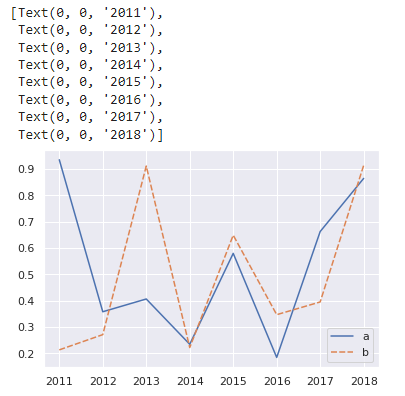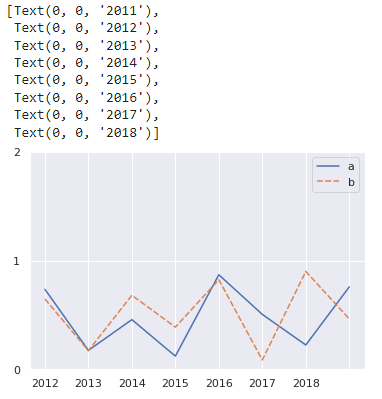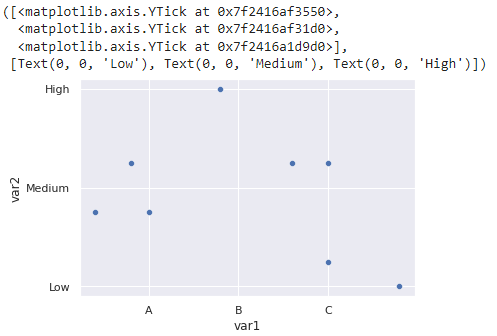# How to Adjust Number of Ticks in Seaborn Plots?

• Last Updated : 19 Dec, 2021

In this article, we will discuss how to adjust the number of ticks in Seaborn Plots. Ticks are the values that are used to show some specific points on the X-Y coordinate, It can be a string or a number. We will see how we can choose an optimal or expand the number of ticks to display on both the x-axis and y-axis.

The Axes.set_xticks() and Axes.set_yticks() functions in axes module of matplotlib library are used to Set the ticks with a list of ticks on X-axis and Y-axis respectively.

Syntax:

For  xticks:

Axes.set_xticks(self, ticks, minor=False)

For  yticks:

Axes.set_yticks(self, ticks, minor=False)

Parameters:

• ticks: This parameter is the list of x-axis/y-axis tick locations.
• minor: This parameter is used whether set major ticks or to set minor ticks

Return value:

This method does not returns any value.

### Example 1: Adjust Number X – Ticks using set_xticks()

In this example, we are setting a number of xticks to the length of data present in dataframe.

## Python3

 `import` `pandas as pd ` `import` `numpy as np ` `import` `seaborn as sns ` `import` `matplotlib.pyplot as plt ` ` `  `sns.``set``(style``=``"darkgrid"``) ` ` `  `# create DataFrame ` `df ``=` `pd.DataFrame({``'a'``: np.random.rand(``8``), ``'b'``: np.random.rand(``8``)}) ` ` `  `# create lineplot ` `g ``=` `sns.lineplot(data``=``df) ` ` `  `# set the ticks first ` `g.set_xticks(``range``(``8``)) ` ` `  `# set the labels ` `g.set_xticklabels([``'2011'``, ``'2012'``, ``'2013'``, ``'2014'``, ` `                   ``'2015'``, ``'2016'``, ``'2017'``, ``'2018'``]) `

Output:### Example 2:  Adjust Number Y – Ticks using set_yticks()

In this example, we are setting a number of yticks to the length of data present in dataframe.

## Python3

 `import` `pandas as pd ` `import` `numpy as np ` `import` `seaborn as sns ` `import` `matplotlib.pyplot as plt ` ` `  `sns.``set``(style``=``"darkgrid"``) ` ` `  `# create DataFrame ` `df ``=` `pd.DataFrame({``'a'``: np.random.rand(``8``), ``'b'``: np.random.rand(``8``)}) ` ` `  `# create lineplot ` `g ``=` `sns.lineplot(data``=``df) ` ` `  `#  set the ticks first ` `g.set_yticks(``range``(``len``(df)``-``5``)) ` ` `  `# set the labels ` `g.set_xticklabels([``'2011'``, ``'2012'``, ``'2013'``, ``'2014'``, ` `                   ``'2015'``, ``'2016'``, ``'2017'``, ``'2018'``]) `

Output:### Example 3:  Adjust Number of X and Y Ticks using xticks() and yticks()

In this example, we are setting the number of specific positions of ticks on x-axis and y-axis

## Python3

 `import` `pandas as pd ` `import` `matplotlib.pyplot as plt ` `import` `seaborn as sns ` ` `  `# create DataFrame ` `df ``=` `pd.DataFrame({``'var1'``: [``25``, ``12``, ``15``, ``14``, ``19``, ``23``, ``25``, ``29``], ` `                   ``'var2'``: [``5``, ``7``, ``7``, ``9``, ``12``, ``9``, ``9``, ``4``]}) ` ` `  `# create scatterplot ` `sns.scatterplot(data``=``df, x``=``'var1'``, y``=``'var2'``) ` ` `  `# specify positions of ticks on x-axis and y-axis ` `plt.xticks([``15``, ``20``, ``25``], [``'A'``, ``'B'``, ``'C'``]) ` `plt.yticks([``4``, ``8``, ``12``], [``'Low'``, ``'Medium'``, ``'High'``]) `

Output:My Personal Notes arrow_drop_up
Recommended Articles
Page :